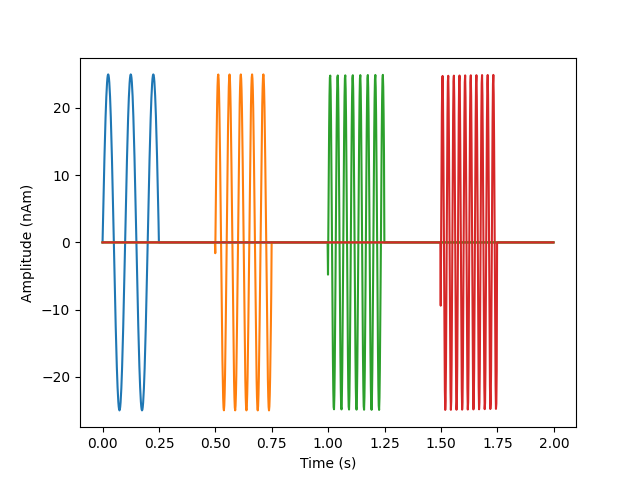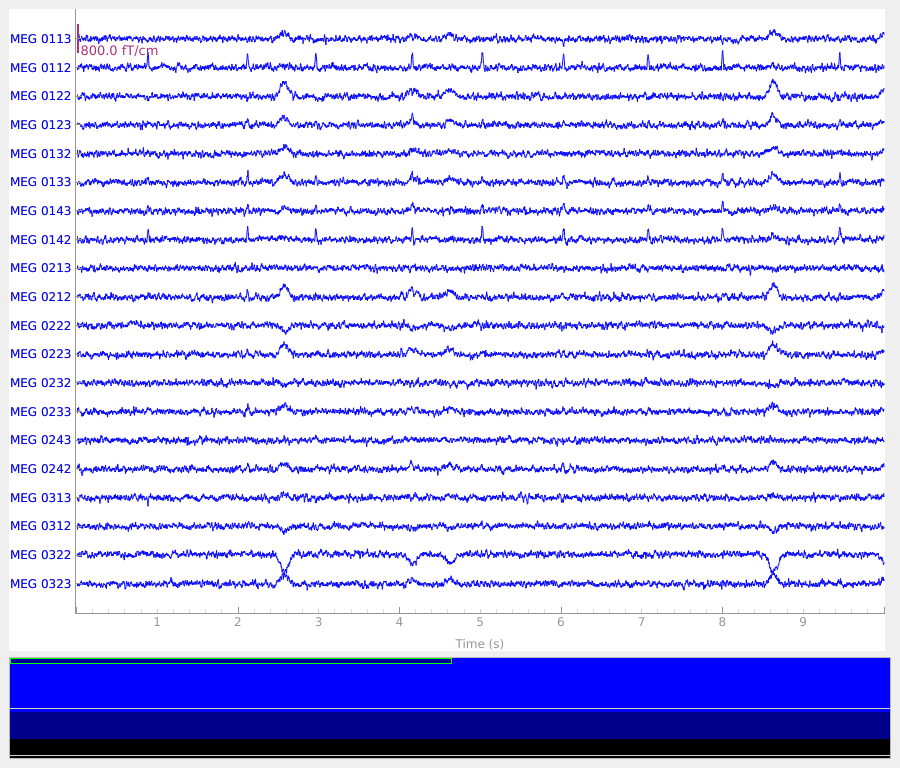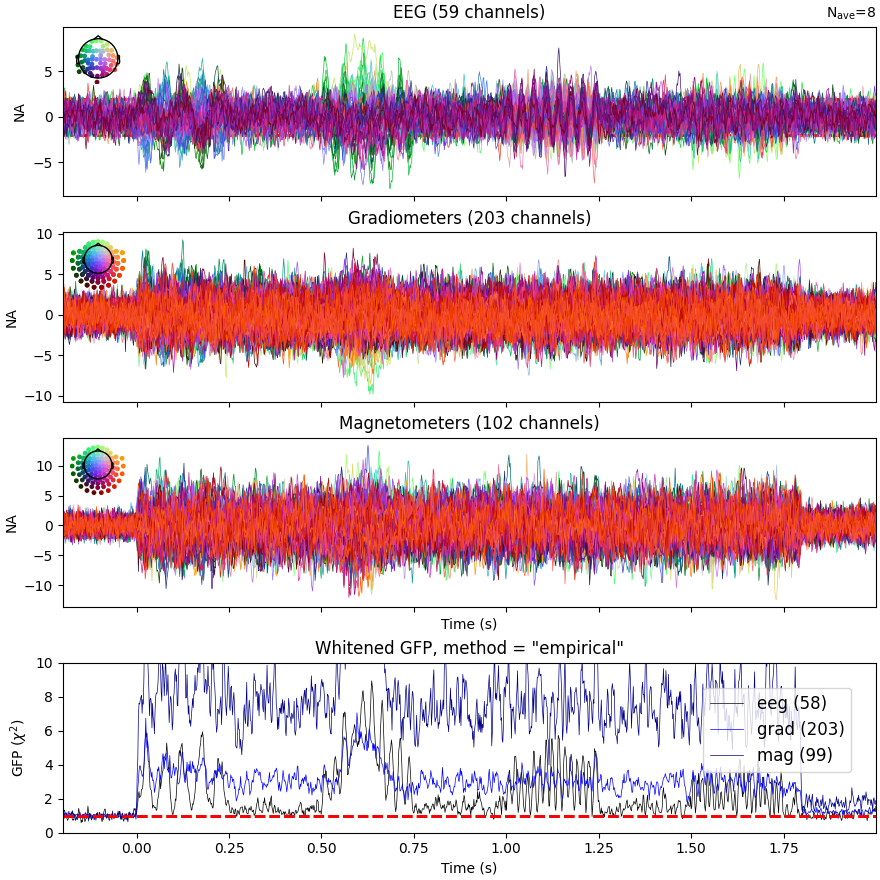# Generate simulated raw data#

This example generates raw data by repeating a desired source activation multiple times.

```# Authors: Yousra Bekhti <yousra.bekhti@gmail.com>
#          Mark Wronkiewicz <wronk.mark@gmail.com>
#          Eric Larson <larson.eric.d@gmail.com>
#
```
```import numpy as np
import matplotlib.pyplot as plt

import mne
from mne import find_events, Epochs, compute_covariance, make_ad_hoc_cov
from mne.datasets import sample
from mne.simulation import (simulate_sparse_stc, simulate_raw,

print(__doc__)

data_path = sample.data_path()
meg_path = data_path / 'MEG' / 'sample'
raw_fname = meg_path / 'sample_audvis_raw.fif'
fwd_fname = meg_path / 'sample_audvis-meg-eeg-oct-6-fwd.fif'

# Load real data as the template
raw.set_eeg_reference(projection=True)
```
```Opening raw data file /home/circleci/mne_data/MNE-sample-data/MEG/sample/sample_audvis_raw.fif...
Read a total of 3 projection items:
PCA-v1 (1 x 102)  idle
PCA-v2 (1 x 102)  idle
PCA-v3 (1 x 102)  idle
Range : 25800 ... 192599 =     42.956 ...   320.670 secs
EEG channel type selected for re-referencing
1 projection items deactivated
```
Measurement date December 03, 2002 19:01:10 GMT MEG Unknown 146 points 204 Gradiometers, 102 Magnetometers, 9 Stimulus, 60 EEG, 1 EOG MEG 2443, EEG 053 EOG 061 Not available 600.61 Hz 0.10 Hz 172.18 Hz PCA-v1 : offPCA-v2 : offPCA-v3 : offAverage EEG reference : off sample_audvis_raw.fif 00:04:38 (HH:MM:SS)

Generate dipole time series

```n_dipoles = 4  # number of dipoles to create
epoch_duration = 2.  # duration of each epoch/event
n = 0  # harmonic number
rng = np.random.RandomState(0)  # random state (make reproducible)

def data_fun(times):
"""Generate time-staggered sinusoids at harmonics of 10Hz"""
global n
n_samp = len(times)
window = np.zeros(n_samp)
start, stop = [int(ii * float(n_samp) / (2 * n_dipoles))
for ii in (2 * n, 2 * n + 1)]
window[start:stop] = 1.
n += 1
data = 25e-9 * np.sin(2. * np.pi * 10. * n * times)
data *= window
return data

times = raw.times[:int(raw.info['sfreq'] * epoch_duration)]
src = fwd['src']
stc = simulate_sparse_stc(src, n_dipoles=n_dipoles, times=times,
data_fun=data_fun, random_state=rng)
# look at our source data
fig, ax = plt.subplots(1)
ax.plot(times, 1e9 * stc.data.T)
ax.set(ylabel='Amplitude (nAm)', xlabel='Time (sec)')
mne.viz.utils.plt_show()
``````Reading forward solution from /home/circleci/mne_data/MNE-sample-data/MEG/sample/sample_audvis-meg-eeg-oct-6-fwd.fif...
Computing patch statistics...
[done]
Computing patch statistics...
[done]
Desired named matrix (kind = 3523) not available
Read MEG forward solution (7498 sources, 306 channels, free orientations)
Desired named matrix (kind = 3523) not available
Read EEG forward solution (7498 sources, 60 channels, free orientations)
Forward solutions combined: MEG, EEG
Source spaces transformed to the forward solution coordinate frame
```

Simulate raw data

```raw_sim = simulate_raw(raw.info, [stc] * 10, forward=fwd, verbose=True)
add_noise(raw_sim, cov, iir_filter=[0.2, -0.2, 0.04], random_state=rng)
raw_sim.plot()
``````Setting up raw simulation: 1 position, "cos2" interpolation
Event information stored on channel:              STI 014
Interval 0.000-2.000 sec
Setting up forward solutions
Computing gain matrix for transform #1/1
Interval 0.000-2.000 sec
Interval 0.000-2.000 sec
Interval 0.000-2.000 sec
Interval 0.000-2.000 sec
Interval 0.000-2.000 sec
Interval 0.000-2.000 sec
Interval 0.000-2.000 sec
Interval 0.000-2.000 sec
Interval 0.000-2.000 sec
10 STC iterations provided
[done]
Adding noise to 366/376 channels (366 channels in cov)
Sphere                : origin at (0.0 0.0 0.0) mm
Source location file  : dict()
Assuming input in millimeters
Assuming input in MRI coordinates

Positions (in meters) and orientations
1 sources
ecg simulated and trace not stored
Setting up forward solutions
Computing gain matrix for transform #1/1
Sphere                : origin at (0.0 0.0 0.0) mm
Source location file  : dict()
Assuming input in millimeters
Assuming input in MRI coordinates

Positions (in meters) and orientations
2 sources
blink simulated and trace stored on channel:      EOG 061
Setting up forward solutions
Computing gain matrix for transform #1/1
```

Plot evoked data

```events = find_events(raw_sim)  # only 1 pos, so event number == 1
epochs = Epochs(raw_sim, events, 1, tmin=-0.2, tmax=epoch_duration)
cov = compute_covariance(epochs, tmax=0., method='empirical',
verbose='error')  # quick calc
evoked = epochs.average()
evoked.plot_white(cov, time_unit='s')
``````Trigger channel has a non-zero initial value of 1 (consider using initial_event=True to detect this event)
Removing orphaned offset at the beginning of the file.
9 events found
Event IDs: 
9 matching events found
Setting baseline interval to [-0.19979521315838786, 0.0] sec
Applying baseline correction (mode: mean)
Created an SSP operator (subspace dimension = 4)
4 projection items activated
Computing rank from covariance with rank=None
Using tolerance 1.1e-14 (2.2e-16 eps * 59 dim * 0.87  max singular value)
Estimated rank (eeg): 58
EEG: rank 58 computed from 59 data channels with 1 projector
Computing rank from covariance with rank=None
Using tolerance 2e-13 (2.2e-16 eps * 203 dim * 4.5  max singular value)
GRAD: rank 203 computed from 203 data channels with 0 projectors
Computing rank from covariance with rank=None
Using tolerance 5.9e-15 (2.2e-16 eps * 102 dim * 0.26  max singular value)
Estimated rank (mag): 99
MAG: rank 99 computed from 102 data channels with 3 projectors
Created an SSP operator (subspace dimension = 4)
Computing rank from covariance with rank={'eeg': 58, 'grad': 203, 'mag': 99, 'meg': 302}
Setting small MEG eigenvalues to zero (without PCA)
Setting small EEG eigenvalues to zero (without PCA)
Created the whitener using a noise covariance matrix with rank 360 (4 small eigenvalues omitted)
```

Total running time of the script: ( 0 minutes 11.235 seconds)

Estimated memory usage: 167 MB

Gallery generated by Sphinx-Gallery